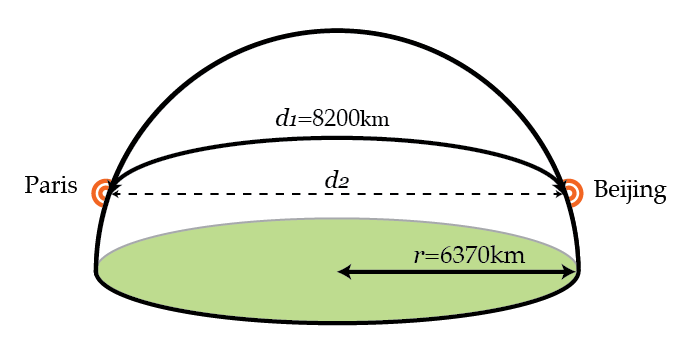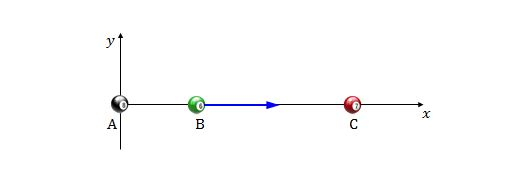Classical Mechanics

# Newton's Law of Gravity - Problem SolvingThe gravity train is a hypothetical idea proposed by Robert Hooke (he of Hooke's law fame) to Isaac Newton in the 1600's. It consists of a simple idea that's hard to implement in practice. Dig a tunnel that runs straight through Earth between two points on the surface. If you can figure out how to remove friction and lower air resistance, you now have a mechanism for extremely efficient and rapid travel between widely separated points. Simply drop something into the tunnel at one end. Gravity will initially pull it downwards through the tunnel, eventually reaching high speeds. Once the object is halfway through the tunnel, gravity will now slow it back down, so you can retrieve the object easily on the other side.

While there are obvious engineering impracticalities, it winds up being pretty amazing how fast a gravity train can get things from point to point without needing fuel. So, let's say we wanted to go from Beijing to Paris using such a gravity train. How long does the trip take assuming a frictionless and drag-free train to the nearest minute?

Earth can be modeled as a sphere of uniform density, with total mass $$6 \times 10^{24}~\mbox{kg}$$ and radius $$6370~\mbox{km}$$. The shortest distance from Beijing to Paris on the surface of Earth is approximately $$8200~\mbox{km}$$.

Details and Assumptions:

• Newton's constant is $$6.67 \times 10^{-11}~\mbox{N m}^2/\mbox{kg}^2$$.
• The mass of the Earth is $$6 \times 10^{24}~\mbox{kg}$$.Consider an $$xy$$-plane in deep space, where three particles are located on the $$x$$-axis, as shown in the above figure. The masses of the three particles are $$m_A=70\text{ kg}, m_B=10\text{ kg}$$ and $$m_C=40\text{ kg},$$ and their respective $$x$$-coordinates are $$x_A=0,$$ $$x_B=5$$ and $$x_C=15$$ in meters. If you move the particle $$B$$ along the $$x$$-axis until its coordinate becomes $$10,$$ approximately how much work is done on particle $$B$$ by you?

Assumptions and Details

• The universal gravitational constant is $$G=6.67 \times 10^{-11} \text{ N}\cdot\text{m}^2\text{/kg}^2.$$

A person standing on the north pole of the earth measures the acceleration due to gravity as $$-9.81~m.s^2$$ by resting a 1 kg block on a spring scale and measuring the force exerted by the scale. What value for the magnitude of the acceleration of gravity in $$m/s^2$$ would a person on the equator measure if they performed this experiment?

Details and assumptions

• You may treat the earth as a perfect sphere of radius 6370 km.
• Take the length of a day to be exactly 24 hours.

An asteroid is falling toward the Earth from deep space. The mass of the asteroid is $$m=6.00 \times 10^9\text{ kg}$$ and that of the Earth is $$5.98 \times 10^{24}\text{ kg}.$$ If it arrived at a point $$9.00 \times 10^8\text{ m}$$ from the center of the Earth, approximately how much work was done by the gravitational force?

Assumptions and Details

• The universal gravitational constant is $$G=6.67 \times 10^{-11} \text{ N}\cdot\text{m}^2\text{/kg}^2.$$

The separation between two particles is $$19.0\text{ m},$$ and the masses of them are $$5.3\text{ kg}$$ and $$2.7\text{ kg}.$$ How much work must one do to triple the separation between the particles?

Assumptions and Details

• The universal gravitational constant is $$G=6.67 \times 10^{-11} \text{ N}\cdot\text{m}^2\text{/kg}^2.$$
×

Problem Loading...

Note Loading...

Set Loading...